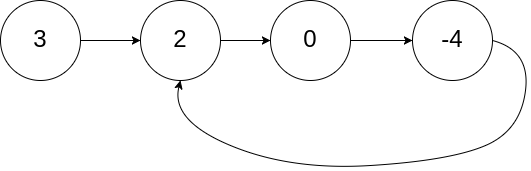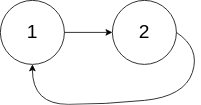# 141. Linked List Cycle / Easy

Given head, the `head` of a linked list, determine if the linked list has a cycle in it.

There is a cycle in a linked list if there is some node in the list that can be reached again by continuously following the `next` pointer. Internally, pos is used to denote the index of the node that tail’s next pointer is connected to. Note that pos is not passed as a parameter.

Return `true` if there is a cycle in the linked list. Otherwise, return `false`.

## Example 1:Input: head = [3,2,0,-4], pos = 1
IOutput: true
IExplanation: There is a cycle in the linked list, where the tail connects to the 1st node (0-indexed).

## Example 2:Input: head = [1,2], pos = 0
Output: true
Explanation: There is a cycle in the linked list, where the tail connects to the 0th node.

## Example 3:Input: head = , pos = -1
Output: false
Explanation: There is no cycle in the linked list.

## Constraints:

• The number of the nodes in the list is in the range [0, 10^4].
• -10^5 <= Node.val <= 10^5
• pos is -1 or a valid index in the linked-list.

# Solution: Floyd’s Cycle Finding Algorithm

## 效能

### Complexity

• Time Complexity: O(N)
• Space Complexity: O(1)

### LeetCode Result

• Runtime: 4 ms
• Memory Usage: 8 MB